×## Formula

An isosceles right triangle has identical edges and a hypotenuse, which can be calculated using the Pythagoras formula.

Here using Pythagoras theorem in the above triangle we get;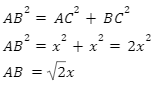Thus the length of the hypotenuse in an isosceles right triangle is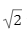times the length of the equal legs.

## Using the standard method for calculating a triangle's area, the area of an isosceles right triangle is found; in this case, the triangle's base and height are its two congruent sides.In ABC, base=x units, altitude(height)=x units.

Therefore the area of an isosceles right triangle is calculated as below;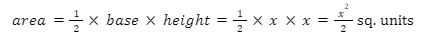## The Perimeter of an Isosceles Right Triangle

The lengths of the edges of any plane shape are added to determine its perimeter. The perimeter of a triangular would be equal to the total of its edges. With these side lengths, the perimeter of a triangle with these sides would be:

In the figure of isosceles right ABC, above the perimeter can be calculated as;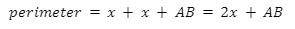## Calculate the area and the perimeter of an isosceles right triangle with the length of the hypotenuse side equal to 15 cm.Solution:In ABC above, applying formulae for area and the perimeter as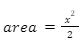and perimeter=2x+AB, we can calculate the required area and the perimeter of the triangle with the length of the hypotenuse 15 cm. First, we shall calculate the value of x using the Pythagoras theorem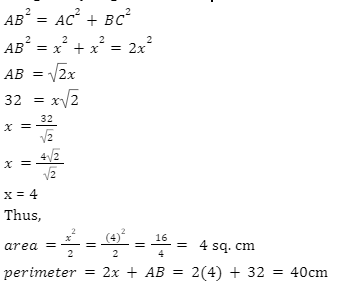## A particular kind of triangle with two equal edges and a right angle is called an isosceles right triangle. The triangle's two equal-length sides are known as the legs, and the third side, which is located across from the right angle, is referred to as the hypotenuse. An isosceles right triangle has advantages that make it helpful in a variety of disciplines, including mathematics, engineering, and building. The Pythagorean theorem, which connects the lengths of the sides to the length of the hypotenuse, and the fact that the angles across from the legs are equal are two of this triangle's noteworthy characteristics. Frequently Asked Questions1. What Makes the Right Isosceles Triangle Unique?The isosceles right triangle combines the characteristics of the right triangle and the isosceles. Two of the sides are the same, two of the angles have the same measure, and one of the angles is 90 degrees.2. In real life, how is the isosceles triangle used?Architectural design often makes use of the isosceles shape. A roof on a house or other structure can have the form of an isosceles, equilateral, or triangular. A church tower can be built using four isosceles cones. Native Americans used the isosceles triangular form to make arrowheads. By using an arrowhead to join the two ends of the two identical sides, an isosceles triangle is formed. 3. What are the various isosceles triangle-related theorems?Ans: An isosceles triangle has angles that are consistent with its congruent sides. This is stated in one of the theories relating to isosceles triangles.

#### Recent Posts